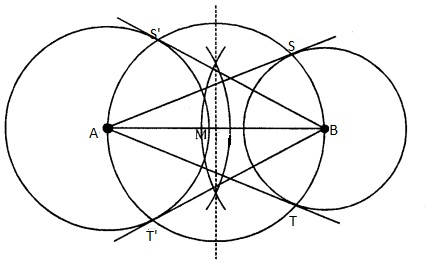# Draw a line segment AB of length 8 cm. Taking A as centre, draw a circle of radius 4 cm and taking B as centre, draw another circle of radius 3 cm. Construct tangents to each circle from the centre of the other circle.

Given:

A line segment AB of length 8 cm. Take two points P and Q on one of its extended diameter each at a distance of 7 cm from its centre.

To do:

We have to draw a line segment AB of length 8 cm. Taking A as centre, draw a circle of radius 4 cm and taking B as centre, draw another circle of radius 3 cm. Construct tangents to each circle from the centre of the other circle.

Solution:Steps of construction:

(i) Draw a line segment $AB = 8\ cm$.

(ii) With centre $A$ and radius $4\ cm$ and with centre $B$ and radius $3\ cm$, draw circles.

(iii) Bisect $AB$ at $M$.

(iv) With centre $M$ and diameter $AB$, draw a circle which intersects the two circles at $S’, T’$ and $S, T$ respectively.

(v) Join $AS, AT, BS’$ and $BT’$.

$AS, AT, BS’$ and $BT’$ are the required tangents.

Updated on: 10-Oct-2022

40 Views